﻿ 基于SSD的马铃薯叶片图像病斑分割算法研究 Research on Leaf Image Disease Spot Segmentation Algorithm Based on SSD Network

Computer Science and Application
Vol. 09  No. 04 ( 2019 ), Article ID: 29711 , 11 pages
10.12677/CSA.2019.94081

Research on Leaf Image Disease Spot Segmentation Algorithm Based on SSD Network

Yanyan Ma1, Guoying Zhang1, Juan Liu2

1China University of Mining & Technology, Beijing, Beijing

2Beijing Information Science & Technology University, Beijing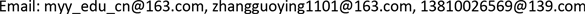Received: Mar. 28th, 2019; accepted: Apr. 8th, 2019; published: Apr. 15th, 2019ABSTRACT

Image-based automatic segmentation of leaf disease spot is the basis for plant disease identification. A deep learning method based on SSD neural network is proposed in order to solve the problem of adaptive extraction of disease spot from uneven image and noise difference images in traditional feature segmentation. The idea of the algorithm is to transform the whole segmentationinto partial segmentation. The depth features are extracted by multi-scale convolution to generate a set of leaf disease spot bounding-boxes, and the disease spot regions of leaf are obtained by non-maximum suppression. Experiments on the image of potato leaves were carried out to obtain the exact boundary of the disease spots in the image. The location accuracy of the disease spot was more than 85%, and the accuracy of boundary segmentation was 80%. Compared with the traditional method of segmentation of disease spot, this method proposes an effective solution for complex background and automatic segmentation of multiple disease spots, which lays a foundation for further leaf disease identification.

Keywords:Disease Spot Segmentation, Deep Learning, Support Vector Machines, Color Model

1中国矿业大学(北京)，北京

2北京信息科技大学，北京Copyright © 2019 by author(s) and Hans Publishers Inc.

This work is licensed under the Creative Commons Attribution International License (CC BY).

http://creativecommons.org/licenses/by/4.0/1. 引言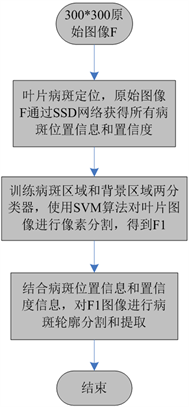Figure 1. Overall framework of the algorithm

2. 基于SSD网络的病斑叶片定位算法

2.1. SSD网络结构

SSD网络是在VGG16网络基础上改进，新增了四个卷积层，基础网络也有微小的改变，网络结构如图2所示。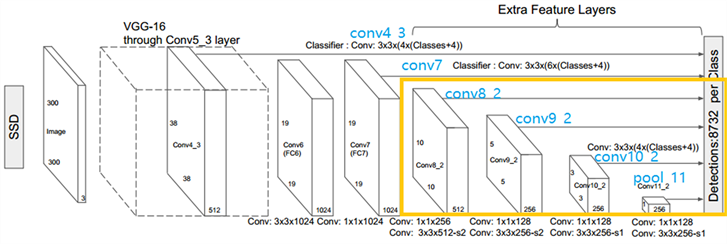Figure2. The framework is an SSD network structure, and the layer used for detection includes four layers of yellow frame area (conv8_2, conv9_2, conv_10_2, pool_11) and two base layers of conv4_3 and conv7 layers

2.2. 基于SSD网络病斑定位模型损失函数

SSD的损失函数公式(1)所示，是分类损失与定位损失加权和。

$L\left(x,c,l,g\right)=\frac{1}{N}\left({L}_{conf}\left(x,c\right)+\alpha {L}_{loc}\left(x,l,g\right)\right)$ (1)

${L}_{loc}\left(x,l,g\right)={\sum }_{i\in Pos}^{N}{\sum }_{m\in \left\{cx,cy,w,h\right\}}{x}_{ij}^{k}smoot{h}_{L1}\left({l}_{i}^{m}-{g}_{j}^{m}\right)$ (2)

${L}_{conf}\left(x,c\right)=-{\sum }_{i\in Pos}^{N}{x}_{ij}^{p}\mathrm{log}\left({c}_{i}^{p}\right)-{\sum }_{i\in Neg}\mathrm{log}\left({c}_{i}^{0}\right)\text{\hspace{0.17em}}\text{where}\text{\hspace{0.17em}}{c}_{i}^{p}=\frac{\mathrm{exp}\left({c}_{i}^{p}\right)}{{\sum }_{p}\mathrm{exp}\left({c}_{i}^{p}\right)}$ (3)

2.3. 基于SSD网络病斑定位模型训练策略

2.3.1. 数据扩增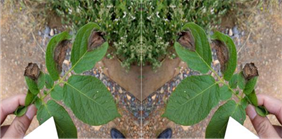Figure 3. The example of horizontal flipping. The image on the left is the original image, and the image on the right is the image after horizontal flip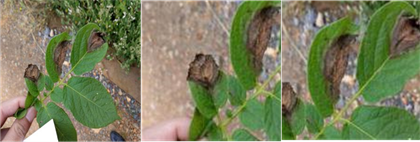Figure 4. The example of random cropping, the left image is the original image, and the middle and right images are random crop images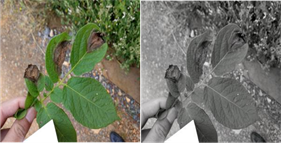Figure 5. The example of color distortion, the left picture is the original picture, and the right picture is the color random transformation result image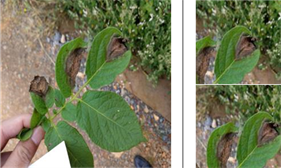Figure 6. The example of a random acquisition block field, the left side of the vertical line is the original picture, and the right side is 2 this random acquisition block domain diagram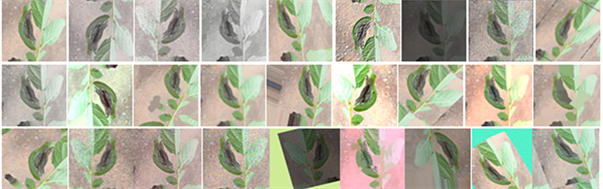Figure 7. The example images of potato data enhancement (the left one is the original image, the rest is the flipped, random cropping and color distortion)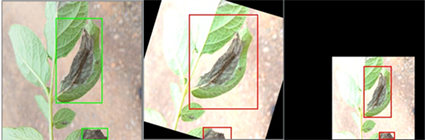Figure 8. The images with bounding boxes, the left image is before the enhancement, and the middle and right images are enhanced. The bounding box is green before it is enhanced and red when it is enhanced

2.3.2. 在conv4_3网络部分进行检测

2.3.3. 使用atrous卷积

2.3.4. 匹配策略

2.4. 叶片病斑定位评价方法

$\text{DR}=\frac{\text{TP}}{\text{TP}+\text{FN}}$ (4)

$\text{FPR}=\frac{\text{FP}}{\text{TP}+\text{FP}}$ (5)

3. 基于SVM叶片图像病斑自动分割提取算法

3.1. SVM图像分割基本原理

3.2. 训练SVM模型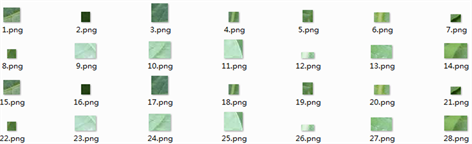Figure 9. The examples of background sample images.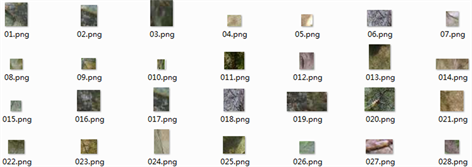Figure 10. The examples of target sample images

4. 实验结果及分析

4.1. 实验数据采集Figure 11. The sample examples of network positioning training sample library

4.2. 实验参数设置

4.3. 实验效果

4.3.1. SSD网络定位算法试验结果与讨论Table 1. Test results statistics of leaf disease spot positioning algorithm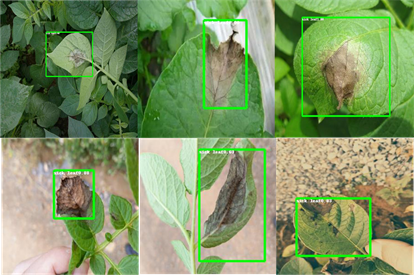Figure 12. Single disease spot leaf positioning effect by SSD network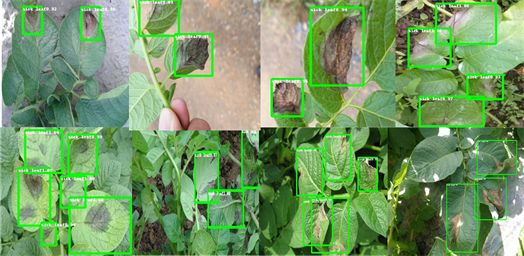Figure 13. Multi-spot leaf positioning effect by SSD network

4.3.2. 病斑分割算法试验结果与讨论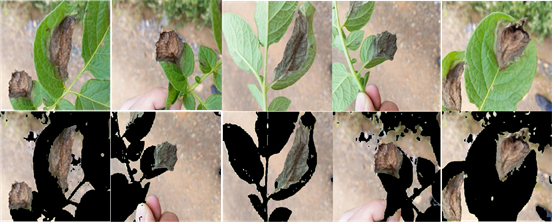Figure 14. Example of pixel segmentation effect directly on the overall leaf image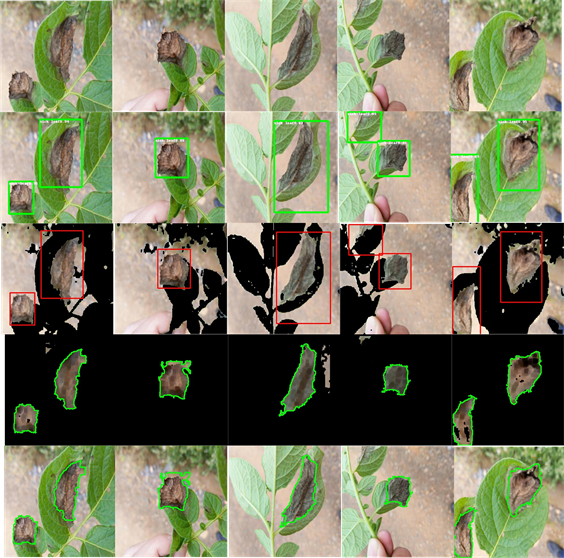Figure 15. The algorithm proposed in this paper performs segmentation of disease spots on leaf images

5. 结论

Research on Leaf Image Disease Spot Segmentation Algorithm Based on SSD Network[J]. 计算机科学与应用, 2019, 09(04): 710-720. https://doi.org/10.12677/CSA.2019.94081

1. 1. Barbedo, J.G.A. (2014) An Automatic Method to Detect and Measure Leaf Disease Symptoms Using Digital Image Processing. Plant Disease, 98, 1709-1716.

2. 2. Ma, J.G. and Barbedo, A. (2013) Digital Image Processing Techniques for Detecting, Quantifying and Classifying Plant Diseases. SpringerPlus, 2, 1-12.

3. 3. 夏勇泉, 李耀斌, 曾莎. 基于HIS颜色空间的植物叶片病斑提取方法[J]. 江苏农业科学, 2015, 43(8): 406-408.

4. 4. 张建华, 韩书庆, 翟治芬, 孔繁涛, 冯鑫, 吴建寨. 改进自适应分水岭方法分割棉花叶部粘连病斑[J]. 农业工程学报, 2018, 34(24): 165-174.

5. 5. 白文斌, 白帆, 贺文文, 等. 基于SVM的高粱叶片病斑图像自动分割提取方法研究[J]. 农学学报, 2014, 4(6): 101-106.

6. 6. 侯兆静, 冯全, 张涛. 基于高斯混合模型的叶片检测分割算法[J]. 计算机应用与软件, 2018, 35(1).

7. 7. Mohanty, S.P., Hughes, D. and Salathé, M. (2016) Using Deep Learning for Image-Based Plant Disease Detection. Frontiers in Plant Science, 7, 1419. https://doi.org/10.3389/fpls.2016.01419

8. 8. Zhang, L., Xia, G.-S., Wu, T., Lin, L. and Tai, X.C. (2016) Deep Learning for Remote Sensing Image Understanding. Journal of Sensors, 2016, Article ID: 7954154.

9. 9. Barbedo, J.G.A. (2016) A Review on the Main Challenges in Automatic Plant Disease Identification Based on Visible Range Images. Biosystems Engineering, 144, 52-60.

10. 10. He, K., Zhang, X., Ren, S. and Sun, J. (2016) Deep Residual Learning for Image Recognition. Proceedings of the IEEE Conference on Computer Vision and Pattern Recognition, Las Vegas, 26 June-1 July 2016, 770-778. https://doi.org/10.1109/CVPR.2016.90

11. 11. Liu, W., Anguelov, D., et al. (2016) SSD: Single Shot MultiBox Detector.

12. 12. 王书舟, 伞冶. 支持向量机的训练算法综述[J]. 智能系统学报, 2008, 3(6): 467-475.

13. 13. 陈佳娟. 基于图像处理和人工智能的植物病害自动诊断技术的研究[D]: [博士学位论文]. 长春: 吉林大学, 2001.

14. 14. 毛罕平, 张艳诚, 胡波. 基于模糊C均值聚类的作物病害叶片图像分割方法研究[J]. 农业工程学报, 2008, 24(9): 136-140.

15. 15. Rafael, C.G., Richard, E.W., 等. 数字图像处理[M]. 第3版. 阮秋琦, 译. 北京: 电子工业出版社, 2013.

16. 16. Szegedy, C., et al. (2014) Going Deeper with Convolu-tions.

17. 17. Russakovsky, O., Deng, J., et al. (2015) Imagenet Large Scale Visual Recognition Challenge. International Journal of Computer Vision, 115, 211-252.

18. 18. Patil, J.K. and Kumar, R. (2012) Feature Extraction of Diseased Leaf Images. Journal of Signal & Image Processing, 3, 60.

19. 19. Al-Hiary, H., Bani-Ahmad, S., Reyalat, M., Braik, M. and Al-Rahamneh, Z. (2011) Fast and Accu-rate Detection and Classification of Plant Diseases. Machine Learning, 14, 5.